JEE  >  VITEEE PCME Mock Test - 3

# VITEEE PCME Mock Test - 3

Test Description

## 125 Questions MCQ Test VITEEE: Subject Wise and Full Length MOCK Tests | VITEEE PCME Mock Test - 3

VITEEE PCME Mock Test - 3 for JEE 2023 is part of VITEEE: Subject Wise and Full Length MOCK Tests preparation. The VITEEE PCME Mock Test - 3 questions and answers have been prepared according to the JEE exam syllabus.The VITEEE PCME Mock Test - 3 MCQs are made for JEE 2023 Exam. Find important definitions, questions, notes, meanings, examples, exercises, MCQs and online tests for VITEEE PCME Mock Test - 3 below.
Solutions of VITEEE PCME Mock Test - 3 questions in English are available as part of our VITEEE: Subject Wise and Full Length MOCK Tests for JEE & VITEEE PCME Mock Test - 3 solutions in Hindi for VITEEE: Subject Wise and Full Length MOCK Tests course. Download more important topics, notes, lectures and mock test series for JEE Exam by signing up for free. Attempt VITEEE PCME Mock Test - 3 | 125 questions in 150 minutes | Mock test for JEE preparation | Free important questions MCQ to study VITEEE: Subject Wise and Full Length MOCK Tests for JEE Exam | Download free PDF with solutions
 1 Crore+ students have signed up on EduRev. Have you?
VITEEE PCME Mock Test - 3 - Question 1

### The perimeter of a sector is p. the area of the sector is maximum when its radius is

Detailed Solution for VITEEE PCME Mock Test - 3 - Question 1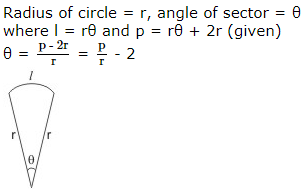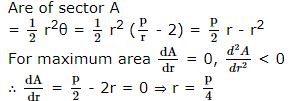VITEEE PCME Mock Test - 3 - Question 2

### Let z,w be complex numbers such that z̅ + iw ̅ = 0 and arg zw = π.Then arg z equals

VITEEE PCME Mock Test - 3 - Question 3

### The area, in square unit, bounded by the curves y = x3, y = x2 and the ordinates x = 1, x = 2 is

VITEEE PCME Mock Test - 3 - Question 4

If (a+ib)(c+id)(e+if)(g+ih) = A+iB, then (a2+b2)(c2+d2)(c2+f2)(g2+h2) =

VITEEE PCME Mock Test - 3 - Question 5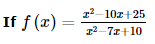for x ≠ 5 and f is continuous at x = 5 then f(5) =

VITEEE PCME Mock Test - 3 - Question 6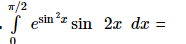Detailed Solution for VITEEE PCME Mock Test - 3 - Question 6

Put sin2x = t

Then sin2x dx = d t ; x = 0, π/2 ⇒ t = 0,1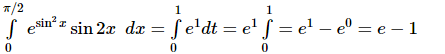VITEEE PCME Mock Test - 3 - Question 7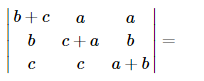VITEEE PCME Mock Test - 3 - Question 8

Let y = | x | + | x − 2 | . Then dy/dx at x = 2 is

VITEEE PCME Mock Test - 3 - Question 9

The solution of the equation (x-y2x)dx=(y-x2y)dy is

VITEEE PCME Mock Test - 3 - Question 10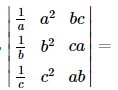Detailed Solution for VITEEE PCME Mock Test - 3 - Question 10

Multiply R1, R2, R3 by a, b, c respectively and divide by abc
Take abc common from C3 so that C1, C3 are identical

VITEEE PCME Mock Test - 3 - Question 11

The solution of dy/dx + 1 = eX+Y is

VITEEE PCME Mock Test - 3 - Question 12

The order of the differential equation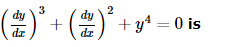VITEEE PCME Mock Test - 3 - Question 13

Integrating factor of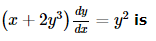VITEEE PCME Mock Test - 3 - Question 14

Differential of x6 w.r. to x3 is equal to

VITEEE PCME Mock Test - 3 - Question 15

Two functions f : R → R , g : R → R are defined as follows :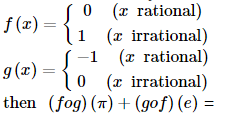VITEEE PCME Mock Test - 3 - Question 16

If ∫(sin 2x - cos 2x)dx = (1/√2) sin (2x-a) + b

VITEEE PCME Mock Test - 3 - Question 17

What is the value of the integral  ∫ ex [ f'x + f x ] dx ?

VITEEE PCME Mock Test - 3 - Question 18

sin[(1/2)cos-1(4/5)] is equal to

VITEEE PCME Mock Test - 3 - Question 19

What is the value of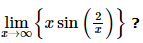VITEEE PCME Mock Test - 3 - Question 20

True statement for
lim x → 0  1 + x − 1 − x2 + 3x − 2 − 3 x is

VITEEE PCME Mock Test - 3 - Question 21

The statement "If x is divisible by 8, then it is divisible by 6" is false if x equals

Detailed Solution for VITEEE PCME Mock Test - 3 - Question 21

A sentence in "If....then..." form is called an implication. The only time when an implication is false is when the "If" part of the sentence is true and the "then" part of the sentence is false. The number 32 makes the first part of the statement true and the second part of the statement false.

VITEEE PCME Mock Test - 3 - Question 22

The system of equation
2x + y = 5
x - 3y = -1
3x + 4y = k
is consistent, when k is

VITEEE PCME Mock Test - 3 - Question 23

If A is a singular matrix then Adj A is

VITEEE PCME Mock Test - 3 - Question 24

Five digit number divisible by 3 is formed using the digits 0, 1 , 2, 3, 4 and 5 without repetition. Total number of such numbers is

Detailed Solution for VITEEE PCME Mock Test - 3 - Question 24

A number is divisible by 3 if and only if the sum of its digits are divisible by 3
Notice that 1 + 2 + 3 + 4 + 5 = 15, which is divisible by 3
The only other way we can have a sum of 5 digits divisible by 3 is to replace the 3 by the 0 making the sum 3 less:
1 + 2 + 0 + 4 + 5 = 12, which is divisible by 3
No other choice of 5 digits can have a sum divisible by 3, because there is no other way to make the sum 12 or 15, and we certainly can't have a sum of 9 or 18
So the number of 5-digit numbers that can be formed from the digits {1,2,3,4,5} is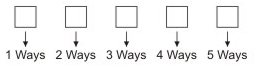Number of ways = 1 x 2 x 3 x 4 x 5 = 120
And the number of 5-digit numbers that can be formed from the digits {1, 2, 0, 4, 5} is figured this way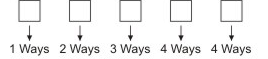Number of ways = 1 x 2 x 3 x 4 x 4 = 96
Total Number of ways = 120 + 96 = 216

VITEEE PCME Mock Test - 3 - Question 25
For a B.D., the parameters n and p are 16 and 1/2 respectively. Then its S.D.σ is equal to
VITEEE PCME Mock Test - 3 - Question 26

If A and B are two events such that P(A ∪ B)   = 0 .65, P(A ∩ B)   = 0.15 then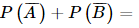VITEEE PCME Mock Test - 3 - Question 27

You are given a box with 20 cards in it. 10 of these cards have the letter 1 printed on them. The other ten have the letter T printed on them. If you pick up 3 cards at random and keep them in the same order, the probability of making the word IIT is

VITEEE PCME Mock Test - 3 - Question 28

If to form a ΔABC it is given that a=5, b=7, sinA=( 3/4 ), then possible triangles are

Detailed Solution for VITEEE PCME Mock Test - 3 - Question 28

Given a = 5, b = 7 and sin A = 3/4
∴ b sin A = 7 x ( 3/4 ) = 21/4 > a (= 5),
Thus is this case no triangle in possible

VITEEE PCME Mock Test - 3 - Question 29

If the A.M. and G.M, of the roots of a quadratic equation in x are p and q respectively, then its equation is

VITEEE PCME Mock Test - 3 - Question 30

If v is the variance and σ is the standard deviation, then

VITEEE PCME Mock Test - 3 - Question 31

Coefficients of correlation between observations (1, 6), (2, 5), (3, 4), (4, 3), (5, 2), (6, 1) is

VITEEE PCME Mock Test - 3 - Question 32

The condition that one root of the equation (1+m2) x2 + 2 cmx + (c2 - a2) = 0 is reciprocal of the other is____

Detailed Solution for VITEEE PCME Mock Test - 3 - Question 32

Let the roots of the equation
(1+m2) x2 + 2 cmx + (c2 - a2) = 0
are α and 1/α .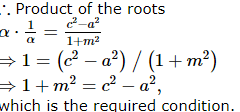VITEEE PCME Mock Test - 3 - Question 33

If | x + 1 | − | x | + 3 | x − 1 || x − 2 | = x + 2 , then solution for − 1 ≤ x ≤ 0 is ___

Detailed Solution for VITEEE PCME Mock Test - 3 - Question 33

When − 1 ≤ x < 0
(x + 1)   − (− x)   + 3 (− x + 1)( − x + 2)   = x + 2
⇒ 3 x2 − 8 x + 5 = 0
⇒ 3 x2  − 3 x − 5 x + 5 = 0
⇒ 3 x − 5 x − 1 = 0
⇒ x = 1 , x = 5 ∕ 3
− 1 ≤ x < 0
Hence, given equation has no solution in − 1 ≤ x < 0. .

VITEEE PCME Mock Test - 3 - Question 34

If cos α, cos β, cos γ are the d.c. of a line, then value of sinα+ sin2β + sin2γ is

VITEEE PCME Mock Test - 3 - Question 35
The Cartesian equation of the plane perpendicular to the line (x - 1)/2 = (y - 3)/-1 = (z - 4)/2 and passing through the origin is
VITEEE PCME Mock Test - 3 - Question 36

If sin2x+sin4x=2sin3x, then x=

VITEEE PCME Mock Test - 3 - Question 37

Let p be a variable point on the ellipse [(x2/a2) + (y2/b2)] = 1 with foci F1 and F2. If A is area of triangle PF1F2, then the maximum value of A is

VITEEE PCME Mock Test - 3 - Question 38

The centre of a circle passing through the points (0, 0), (1, 0) and touching the circle x2 + y2 = 9 is

VITEEE PCME Mock Test - 3 - Question 39

If a,b,c are three non-coplanar vectors, then [a+b,b+c,c+a]=-

VITEEE PCME Mock Test - 3 - Question 40

i x (a x i)+j x (a x j)+k x (a x k)=

VITEEE PCME Mock Test - 3 - Question 41

In a circuit containing an inductance of zero resistance, the emf of the applied A.C. voltage leads the current by

VITEEE PCME Mock Test - 3 - Question 42

In photo electric effect the stopping potential is

Detailed Solution for VITEEE PCME Mock Test - 3 - Question 42

In the photoelectric effect is that the stopping potential is a function of the frequency of the incident light. As shown in Figure, there is even a cutoff frequency ν0 below which there is no photoelectric effect occurs, regardless of the intensity of the light.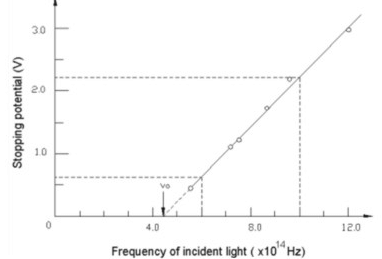A plot of stopping potential vs. frequency of incident light (Cathode: Sodium ; Cutoff frequency νo =4.39x 1014 Hz
Hence option (A) is correct.

VITEEE PCME Mock Test - 3 - Question 43

Which of the following metal thermionically emit an electron at a relatively lowest temperature among them?

VITEEE PCME Mock Test - 3 - Question 44

A metal foil of negligible thickness is introduced between two plates of a capacitor of capacitance C at the centre. The new capacitance of the capacitor will be

VITEEE PCME Mock Test - 3 - Question 45

A steady current of 1.5A flows through a copper voltameter for 10 min. If the electrochemical equivalent of copper is 30 x 10-5 gC-1, the mass of copper deposited on the electrode will be

VITEEE PCME Mock Test - 3 - Question 46

If sky wave with a frequency of 50 MHz is incident on D-region at an angle of 300 then angle of refraction is

VITEEE PCME Mock Test - 3 - Question 47

In a metre bridge, the balancing length from the left end (standard resistance of one ohm is in the right gap) is found to be 20 cm. The value of the unknown resistance is

VITEEE PCME Mock Test - 3 - Question 48

In a Wheatstone's bridge, all the four arms have equal resistance R. If resistance of the galvanometer arm is also R, then equivalent resistance of the combination is

VITEEE PCME Mock Test - 3 - Question 49

Four two ohm resistors are connecte together along the edges of a square. A 10V battery of negligible internal resistance is connected across a pair of the diagonally opposite corners of the square. The power dissipated in the circuit is

VITEEE PCME Mock Test - 3 - Question 50

Which of the following does not obey the ohm's law?

VITEEE PCME Mock Test - 3 - Question 51

Particle nature and wave nature of electromagnetic waves and electrons be shown by

VITEEE PCME Mock Test - 3 - Question 52

A choke coil has

VITEEE PCME Mock Test - 3 - Question 53

R, L and C represent the physical quantities resistance, inductance and capacitance respectively. Which one of the following combinations has dimension of frequency?

VITEEE PCME Mock Test - 3 - Question 54

A metal disc of radius 100 cm is rotated at a constant angular speed of 60 rad/s in a plane at right angles to an external field of magnetic induction 0.05 Wb/m2. The emf induced between the centre and a point on the rim will be

VITEEE PCME Mock Test - 3 - Question 55

If the electric flux entering and leaving an enclosed surface respectively is φ1 and φ2 , the electric charge inside the surface will be

VITEEE PCME Mock Test - 3 - Question 56

Electromagnetic radiation of highest frequency is

VITEEE PCME Mock Test - 3 - Question 57

A hollow metal sphere of radious 10 cm is charged such that the potential on its surface is 80V. The potential at the centre of the sphere is

VITEEE PCME Mock Test - 3 - Question 58

Equal charges are given to two spheres of different radii. The potential will be

VITEEE PCME Mock Test - 3 - Question 59

Two equal and unlike charges places 3 cm apart in air, attract each other with a force of 40 N. The magnitude of each charge in micro coulomb is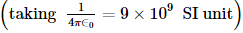VITEEE PCME Mock Test - 3 - Question 60

A 2 μ F condenser is charged to 500V and then its plates are joined through of consistance. The heat produced in the resistance in joules is

VITEEE PCME Mock Test - 3 - Question 61

The figure shows a charge q placed inside a cavity in an uncharged conductor. Now if an external electric field is switched on: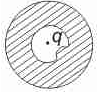VITEEE PCME Mock Test - 3 - Question 62

A straight wire carrying current I is turned into a circular loop. If the magnitude of magnetic moment associated with it in M.K.S. unit is M, the length of wire will be

VITEEE PCME Mock Test - 3 - Question 63

Which of the following is more effective in inducing nuclear fission

VITEEE PCME Mock Test - 3 - Question 64

A radioactive element has a half life of 15 years. The fraction that will decay in 30 years is

VITEEE PCME Mock Test - 3 - Question 65

The photoelectric threshold of a certain metal is 30 Å. If the radiation of 2000 Å is incident on the metal

VITEEE PCME Mock Test - 3 - Question 66

Mass - energy equation was proposed by

VITEEE PCME Mock Test - 3 - Question 67

Light of wavelength λ and photon energy 2 eV falling on a metal surface produces photoelectrons of maximum velocity ν. If λ is decreased by 25% and the maximum velocity is doubled, the work function of metal (in eV) is

VITEEE PCME Mock Test - 3 - Question 68

A glass slab is placed in the path of convergent light. The point of convergence of light

VITEEE PCME Mock Test - 3 - Question 69

The image formed by an objective of a compound microscope is

VITEEE PCME Mock Test - 3 - Question 70

Application of a forward bias to a p-n junction

VITEEE PCME Mock Test - 3 - Question 71

The element that can be used as acceptor impurity to dope silicon is

VITEEE PCME Mock Test - 3 - Question 72

In a common-base amplifier, the phase difference between the input signal voltage and output voltage is

Detailed Solution for VITEEE PCME Mock Test - 3 - Question 72

A common-emitter amplifier is shown in the figure (A).
In this Circuit , the output signal is an amplified version of the input signal and is 180 degrees out of phase with the input signal.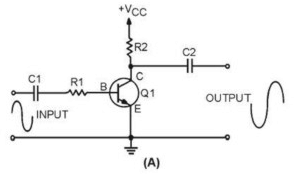Common emitter Amplifier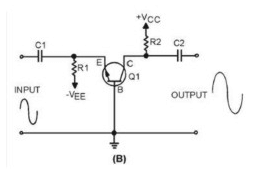Common Base Amplifier

Figure (B) is a common-base amplifier. In this circuit the output signal is an amplified version of the input signal and is in phase with the input signal. In both of these circuits, the output signal is controlled by the base-to-emitter bias. As this bias changes (because of the input signal) the current through the transistor changes.

VITEEE PCME Mock Test - 3 - Question 73

A half-wave rectifier is being used to rectify an alternating voltage of frequency 50 Hz. The number of pulses of rectified current obtained in one second is

VITEEE PCME Mock Test - 3 - Question 74

The intrinsic semiconductor becomes an insulator at

VITEEE PCME Mock Test - 3 - Question 75

The difference in the variation of resistance with temperature in a metal and a semiconductor arises essentially due to the difference in the

VITEEE PCME Mock Test - 3 - Question 76

The bond that exists in a semiconductor is

VITEEE PCME Mock Test - 3 - Question 77

A wave front travels

VITEEE PCME Mock Test - 3 - Question 78

Which of the following property of light waves is not observed in sound waves?

VITEEE PCME Mock Test - 3 - Question 79

If the phase differenc between light waves from two slits of Young's double slit experiment is π radian, then the central fringe will be

VITEEE PCME Mock Test - 3 - Question 80

Bubble's of colourless soap solution appear coloured in sun light. This is due to

VITEEE PCME Mock Test - 3 - Question 81

The reagent required to convert propene to 1-Propanol is

VITEEE PCME Mock Test - 3 - Question 82

In nucleophilic substitution reactions, the reactivity of carbonyl compounds follows the order

VITEEE PCME Mock Test - 3 - Question 83

The most reactive compound towards formation of cyanohydrin on treatment with KCN followed by acidification is

VITEEE PCME Mock Test - 3 - Question 84

In which of the following reactions, both oxidised and reduced forms of the same compound are obtained?

VITEEE PCME Mock Test - 3 - Question 85

Wurtz reaction involves the interaction of alkyl halides in dry ether with

VITEEE PCME Mock Test - 3 - Question 86

Ozonolysis of C₇H₁₄ gave 2-methyl-3-pentanone toluene. The alkene is

VITEEE PCME Mock Test - 3 - Question 87

In the following reaction, which choice has value twice that of the equivalent mass of the oxidising agent SO₂ + H₂O → 3S + 2H₂O

VITEEE PCME Mock Test - 3 - Question 88

The enzyme present in saliva is

VITEEE PCME Mock Test - 3 - Question 89

The volume in litres of CO2 liberated at STP when 10 grams of 90% pure limestone is heated completely is

Detailed Solution for VITEEE PCME Mock Test - 3 - Question 89

Limestone (CaCO3) has a molar mass of = 100 g/mol
So, 10 gm of 100% pure CaCO3 would = 10 gm of CaCO3
90% pure CaCO3 means, each 10 gm of sample will have, = ( 90/100 ) x 10 = 9 gm CaCO3
So, number of moles of CaCO3 = given mass/ molar mass = 9/100 = 0.09
Now, we have the reaction,
CaCO3 → CaO + CO2
When 1 mole of CaCO3 yields 1 moles of CO2
0.09 moles of CaCO3 will yield 0.09 moles of CO2
1 mole of CO2 = 22.4 liters (at STP)
So, total volume = 22.4 x 0.09 = 2.016 liters

VITEEE PCME Mock Test - 3 - Question 90

Which of the following biomolecule is insoluble in water?

VITEEE PCME Mock Test - 3 - Question 91

Pick out the one which does not belong to family of enzymes.

VITEEE PCME Mock Test - 3 - Question 92

Acetyl chloride can not be obtained by treating acetic acid with

VITEEE PCME Mock Test - 3 - Question 93

A colourless liquid at room temperature reacts with soda lime to form sodium salt of carboxylic acid and ammonia gas.The liquid is

VITEEE PCME Mock Test - 3 - Question 94

Rancidity of butter is due to the formation of

VITEEE PCME Mock Test - 3 - Question 95

Chemical equilibrium is dynamic in nature, because

Detailed Solution for VITEEE PCME Mock Test - 3 - Question 95

The two opposite processes, forward reaction and reverse reaction, keep on occurring in it all the time but at exactly equal rates (or equal speeds)
A chemical reaction does not stop after reaching the equilibrium state, only the rate of the forward and the reverse reactions become equal at the equilibrium state

VITEEE PCME Mock Test - 3 - Question 96

In a reversible reaction, the catalyst

VITEEE PCME Mock Test - 3 - Question 97

Latent heat of vaporisation of a liquid at 500 K and 1 atm pressure is 10.0 kcal/mol. What will be the change in internal energy (ΔE) of 3 mol of liquid at same temperature?

Detailed Solution for VITEEE PCME Mock Test - 3 - Question 97

Vaporization of 3 moles of H2O vapors is:
3H2O(l) → 3H2O(g)
Δn = 3 - 0 = 3
Therefore,
ΔU = ΔH - ΔnRT
= (3 x10) - 3 (0.002) (500) = 27
change in internal energy is:
ΔU = 27 Kcal

VITEEE PCME Mock Test - 3 - Question 98

The minimum energy required by molecules to react is called

VITEEE PCME Mock Test - 3 - Question 99

Given that
2C s + 2O2 g → 2CO2 g , Δ H = − 787 k J
H2 g + 12O2 g → H2O l , Δ H = − 286 k J
C2H2 g + 2 1/2 O2 g → 2CO2 + H2O l , Δ H = − 1301 k J
Heat of formation of acetylene is

VITEEE PCME Mock Test - 3 - Question 100

The primary and secondary valencies of chromium in the complex ion, dichlorodioxalatochromium (III) are respectively

VITEEE PCME Mock Test - 3 - Question 101

Which one is false in the following statements ?

VITEEE PCME Mock Test - 3 - Question 102

VITEEE PCME Mock Test - 3 - Question 103

In which of the following complex the oxidation number of the central metal atom is zero?

VITEEE PCME Mock Test - 3 - Question 104

In the diazotisation of aniline with sodium nitrite and hydrochloric acid, the excess of hydrochloric acid is used primarily to

VITEEE PCME Mock Test - 3 - Question 105

Which of the following is produced by reaction of RCN in sodium and alcohol ?

VITEEE PCME Mock Test - 3 - Question 106

The pH of aqueous KCl solution is 7.0. This solution was electrolysed for a few seconds using Pt electrodes. Which of the following is correct?

VITEEE PCME Mock Test - 3 - Question 107

In a galvanic cell, the electrons flow from

VITEEE PCME Mock Test - 3 - Question 108

What is the standard cell potential for the cell? Zn ; Zn2+(1M) ∥ Cu2+(1M) ; Cu (E0 for Zn2+∕Zn = −0.76 ; E0for Cu2⁺∕Cu = +0.34)

VITEEE PCME Mock Test - 3 - Question 109

When diethyl ether is treated with excess of Cl₂ in the presence of sunlight, then the product formed is

VITEEE PCME Mock Test - 3 - Question 110

When the densities of the ore particles and gangue particles are different, then the ore is concentrated by

VITEEE PCME Mock Test - 3 - Question 111

If the pressure and absolute temperatre of 2 litres of CO₂ are doubles, the volume of CO₂ would become

VITEEE PCME Mock Test - 3 - Question 112

Gun metal is an alloy of

VITEEE PCME Mock Test - 3 - Question 113

When iron or zinc is added to CuSO₄ solution, copper is precipitated. It is due to

VITEEE PCME Mock Test - 3 - Question 114

Maximum enolisation takes place of:

VITEEE PCME Mock Test - 3 - Question 115

The most stable hydride is

VITEEE PCME Mock Test - 3 - Question 116

When concentrated H₂SO₄ is heated with dry KNO₃, brown fumes evolve. These fumes are due to

VITEEE PCME Mock Test - 3 - Question 117

The ratio of cationic radius to anionic radius in an ionic crystal is greater than 0.732. Its co-ordination number is

VITEEE PCME Mock Test - 3 - Question 118

The separation of colloidal particles from those of molecular dimensions is known as

VITEEE PCME Mock Test - 3 - Question 119

When calomel reacts with ammonium hydroxide, we get

VITEEE PCME Mock Test - 3 - Question 120

Finely divided iron combines with CO to give

VITEEE PCME Mock Test - 3 - Question 121

Fill in the blank with appropriate word.
Soft minded individuals are........to embrace all kinds of superstitions.

VITEEE PCME Mock Test - 3 - Question 122

Find the antonym of CALLOUS

VITEEE PCME Mock Test - 3 - Question 123

Find the Synonym of COLOSSUS

VITEEE PCME Mock Test - 3 - Question 124

Improve the sentence by choosing best alternative for capitalised part of the sentence.

Poor Tom LAID in the shade of a tree before he could walk further.

VITEEE PCME Mock Test - 3 - Question 125

Fill in the blanks with the most suitable idiom/phrase.

Let us now _____ and work for the advancement of the country .

## VITEEE: Subject Wise and Full Length MOCK Tests

1 videos|3 docs|73 tests
 Use Code STAYHOME200 and get INR 200 additional OFF Use Coupon Code
Information about VITEEE PCME Mock Test - 3 Page
In this test you can find the Exam questions for VITEEE PCME Mock Test - 3 solved & explained in the simplest way possible. Besides giving Questions and answers for VITEEE PCME Mock Test - 3, EduRev gives you an ample number of Online tests for practice

## VITEEE: Subject Wise and Full Length MOCK Tests

1 videos|3 docs|73 tests